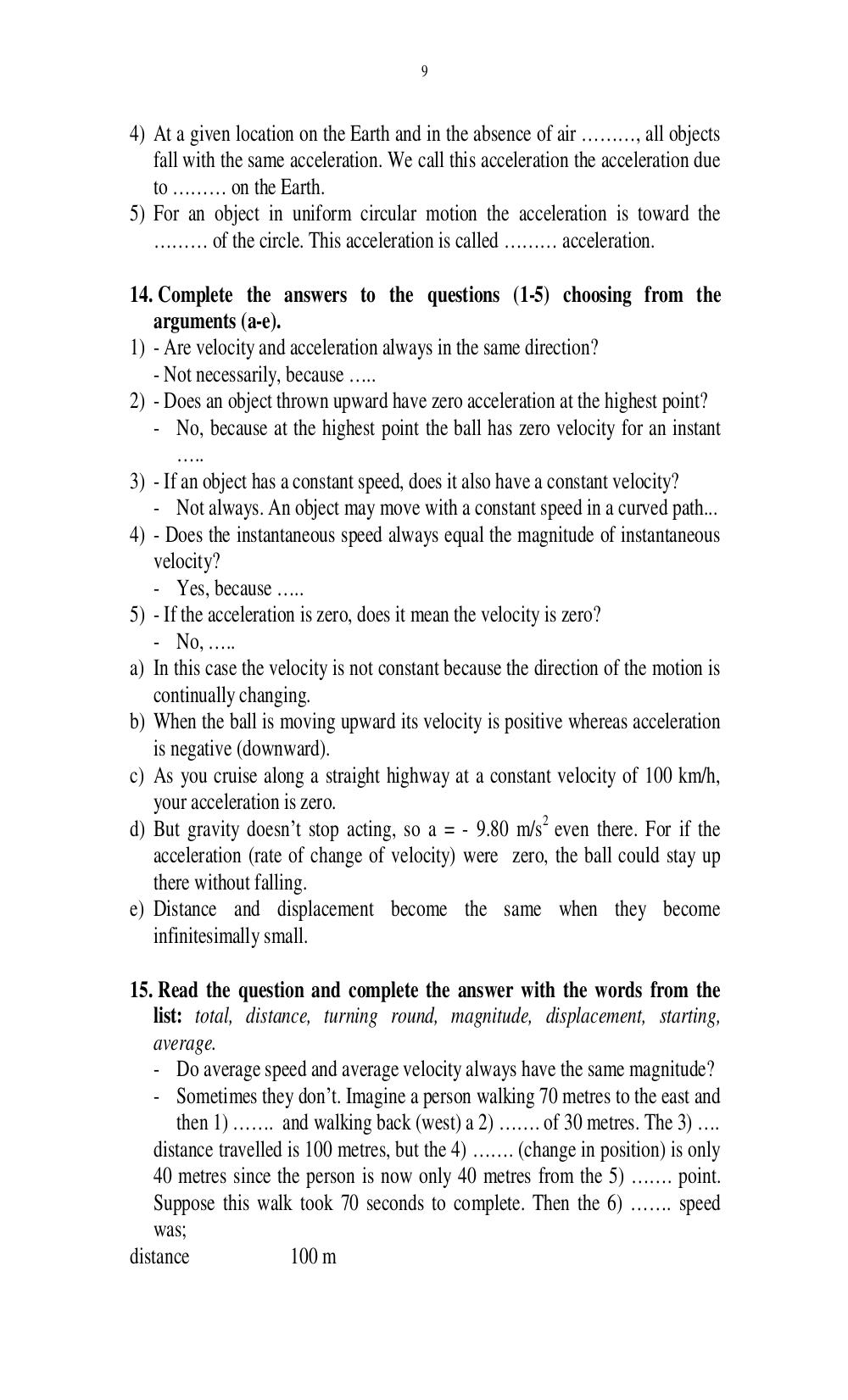# Английский язык. Ильичева Н.А - 9 стр.

Составители:

Рубрика:

• ## Иностранный язык

9
4) At a given location on the Earth and in the absence of air ………, all objects
fall with the same acceleration. We call this acceleration the acceleration due
to …… on the Earth.
5) For an object in uniform circular motion the acceleration is toward the
……… of the circle. This acceleration is called …… acceleration.
14. Complete the answers to the questions (1-5) choosing from the
arguments (a-e).
1) - Are velocity and acceleration always in the same direction?
- Not necessarily, because ..
2) - Does an object thrown upward have zero acceleration at the highest point?
- No, because at the highest point the ball has zero velocity for an instant
..
3) - If an object has a constant speed, does it also have a constant velocity?
- Not always. An object may move with a constant speed in a curved path...
4) - Does the instantaneous speed always equal the magnitude of instantaneous
velocity?
- Yes, because ..
5) - If the acceleration is zero, does it mean the velocity is zero?
- No, ..
a) In this case the velocity is not constant because the direction of the motion is
continually changing.
b) When the ball is moving upward its velocity is positive whereas acceleration
is negative (downward).
c) As you cruise along a straight highway at a constant velocity of 100 km/h,
d) But gravity doesnt stop acting, so a = - 9.80 m/s
2
even there. For if the
acceleration (rate of change of velocity) were zero, the ball could stay up
there without falling.
e) Distance and displacement become the same when they become
infinitesimally small.
15. Read the question and complete the answer with the words from the
list: total, distance, turning round, magnitude, displacement, starting,
average.
- Do average speed and average velocity always have the same magnitude?
- Sometimes they dont. Imagine a person walking 70 metres to the east and
then 1) ……. and walking back (west) a 2) ……. of 30 metres. The 3) .
distance travelled is 100 metres, but the 4) ……. (change in position) is only
40 metres since the person is now only 40 metres from the 5) ……. point.
Suppose this walk took 70 seconds to complete. Then the 6) ……. speed
was;
distance 100 m9

4) At a given location on the Earth and in the absence of air ………, all objects
fall with the same acceleration. We call this acceleration the acceleration due
to ……… on the Earth.
5) For an object in uniform circular motion the acceleration is toward the
……… of the circle. This acceleration is called ……… acceleration.

14. Complete the answers to the questions (1-5) choosing from the
arguments (a-e).
1) - Are velocity and acceleration always in the same direction?
- Not necessarily, because …..
2) - Does an object thrown upward have zero acceleration at the highest point?
- No, because at the highest point the ball has zero velocity for an instant
…..
3) - If an object has a constant speed, does it also have a constant velocity?
- Not always. An object may move with a constant speed in a curved path...
4) - Does the instantaneous speed always equal the magnitude of instantaneous
velocity?
- Yes, because …..
5) - If the acceleration is zero, does it mean the velocity is zero?
- No, …..
a) In this case the velocity is not constant because the direction of the motion is
continually changing.
b) When the ball is moving upward its velocity is positive whereas acceleration
is negative (downward).
c) As you cruise along a straight highway at a constant velocity of 100 km/h,
d) But gravity doesn’t stop acting, so a = - 9.80 m/s2 even there. For if the
acceleration (rate of change of velocity) were zero, the ball could stay up
there without falling.
e) Distance and displacement become the same when they become
infinitesimally small.

15. Read the question and complete the answer with the words from the
list: total, distance, turning round, magnitude, displacement, starting,
average.
- Do average speed and average velocity always have the same magnitude?
- Sometimes they don’t. Imagine a person walking 70 metres to the east and
then 1) ……. and walking back (west) a 2) ……. of 30 metres. The 3) ….
distance travelled is 100 metres, but the 4) ……. (change in position) is only
40 metres since the person is now only 40 metres from the 5) ……. point.
Suppose this walk took 70 seconds to complete. Then the 6) ……. speed
was;
distance               100 m